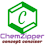Welcome to Chem Zipper.com......: ATOMIC MASS AND MOLECULAR MASS:

## Tuesday, May 21, 2019

### ATOMIC MASS AND MOLECULAR MASS:

ATOM AND MOLECULES:
Atom: Atom is the ultimate electrically neutral, made up of fundamental particle (Electron, neutron, Proton) which shows the characteristic properties of the element and exist freely in a chemical reaction.
Molecules: A molecule is the smallest particle made up of one or more than one atom in a definite ratio having stable and independent existence e.g.H2 ,O2 He HCl , CaCO3 etc.
(1) Atomic Mass: As atoms are very tiny particles, their absolute masses are difficult to measure. However it is possible to determine the relative masses of different atoms if small unit of mass is taken as standard there is three conventions given as.
(1) This standard was mass of one atom of hydrogen and taken as unity.
(2)  And also mass of one atom is 1/16th part of oxygen atom
(3) And now mass of one atom is 1/12th part of C-12 atom.
The atomic mass of an element can be defined as the number which indicates how many times the mass of one atom of the element is heavier in comparison to the mass of one atom of hydrogen.
(2) Atomic Mass Unit:
(1) The quantity 1/12Th mass of an atom of carbon-12 is known as the atomic mass unit and is abbreviated as amu.
(2) The actual mass of one atom of carbon-12 is –
Determination of atomic mass:
(1)  Applying Dulong and Petit’s law.
(2)  Cannizzaro’s methods
(3)  By mitscherlich’s law of isomorphism.
(4)  By measurement of V.D. of volatile chloride or bromide.
(1) Dulong & Petits Law: The product of specific heat of pure element and atomic mass of the element is equal to 6.4.
NOTE:
(1) But this law is not applicable to lighter element like boron, carbon, silicon. To obtain correct atomic mass of element first of all equivalent mass of the element is known by any other method and their atomic mass = eq. weight ´ valency
(2) In which valency has whole number value which can be deduced by dividing approximate by equivalent mass.  Dulongs and Petit’s Law:  Atomic mass ´ specific heat = 6.4

ILLUSTRATIVE EXAMPLE(1): The specific heat of a metal of atomic mass 32 is likely to be:
(A)  0.25                                                                (B)  0.24
(C)  0.20                                                                (D)  0.1
SOLUTION:
ILLUSTRATIVE EXAMPLE(2): On dissolving 6 gm of metal in sulphuric acid, 13.53g of the metal sulphate was formed. The specific heat of metal is 0.057 Cal/g. What is equivalent mass of metal, valency and exact atomic mass?
SOLUTION:
(2)  Cannizzaro’s methods:
If an element has several compound with other same or different elements of known atomic mass then the compound that has minimum presence of former element indicate the atomic mass of former element.
Procedure:
(1)  First of all the molecular mass of all compounds known by applying
V.D ´ 2 = mol. weight
(2)  By analysis the presence of the desired element in each compound is known.
(3)  The mass that is lowest among all the compound indicate the atomic mass
of that element
ILLUSTRATIVE EXAMPLE (3): Estimate the atomic mass of nitrogen given that vapour density of NH3 = 8.5, Nitrous oxide = 22, Nitric oxide = 15, Nitrogen peroxide = 23, Nitrogen
trioxide = 38.
SOLUTION:
(3)  Law of Isomorphism
When two or more compound forms similar type of crystals or able to form mixed crystals, they are known as isomorphs. For examples: MgSO4.7H2O, ZnSO4.7H2O and FeSO4.7H2O are isomorphs of each other as their crystals posses same shape.
According to Mitscherlich [year 1819]. The valency of elements that are similarly placed to that of other elements in their isomorphs are always same.
In the above example Fe, Zn and Mg have same valency  and equal ratio of water molecule in each isomorphs.
If equivalent mass of one element is known then atomic mass can be calculated by knowing the valency of other isomorphs key element.
ILLUSTRATIVE EXAMPLE(4): Which pair of the following substances is said to be isomorphous?
(A) White vitriol and blue vitriol        (B) Epsom salt and Glauber’s salt
(C) Blue vitriol and Glauber’s salt      (D) White vitriol and Epsom salt
SOLUTION:   Epsom salt (MgSO4.7H2O) and White vitriol (ZnSO4.7H2O) contains divalent cation Mg2+ and Zn2+ and same number of water molecules as water of crystallization which hold criteria for isomorphism. Hence (D) is correct.
(4)  Atomic mass from vapour density of a chloride:
The following steps are involved in this method
(1) Vapour density of chloride of the element is determined
(2) Equivalent mass of the element is determined
(3) Let the valency of the element be x. The formula of its chloride will be MClX
ILLUSTRATIVE EXAMPLE(5): One gram of chloride was found to contain 0.835g of chlorine. Its vapour density is 85. Calculate its molecular formula
SOLUTION:

TRY YOURSELF:

Exercise (1): Two oxides of a metal contain 63.2% and 69.62% of the metal. The specific heat of the metal is 0.117. What is the formula of the two oxides?
Exercise (2): 1 g of a metal which has specific heat of 0.06 combines with oxygen to form 1.08 g of oxide. What is the mass of M?
Exercise (3): The chloride of a solid metallic element contains 57.89% by mass of the element. The specific heat of the element is 0.0324 cal deg-1g-1. Calculate the exact atomic mass of the element.
Exercise (4): White vitriol (hydrated sulphite) is isomorphous with MgSO4.7H2O. White vitriol contains 22.95% zinc and 43.9% of water of crystallization. Find the atomic mass of zinc.
Exercise (5): Two oxides of metals A and B are isomorphous. The metal A whose atomic mass is 52, forms a chloride whose vapour density is 79. The oxide of the metal B contains 47.1% oxygen. Calculate the atomic mass of B.

1.1.